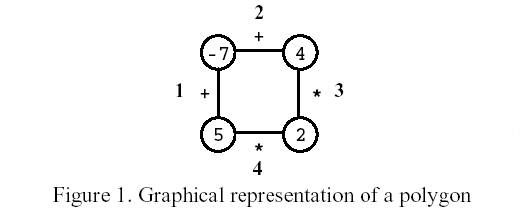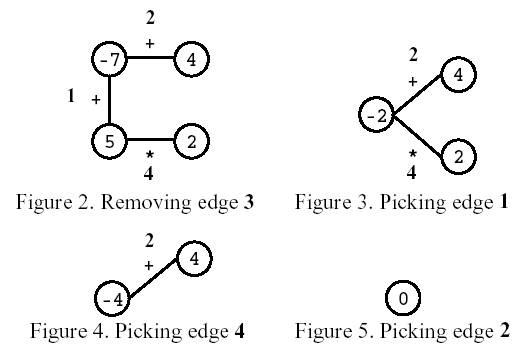# POJ 1179 [IOI1998]Polygon 题解【区间DP】

正常的拆环为链的环形->区间DP。

## Description

Polygon is a game for one player that starts on a polygon with N vertices, like the one in Figure 1, where N=4. Each vertex is labelled with an integer and each edge is labelled with either the symbol + (addition) or the symbol * (product). The edges are numbered from 1 to N.On the first move, one of the edges is removed. Subsequent moves involve the following steps:
·pick an edge E and the two vertices V1 and V2 that are linked by E; and
·replace them by a new vertex, labelled with the result of performing the operation indicated in E on the labels of V1 and V2.
The game ends when there are no more edges, and its score is the label of the single vertex remaining.
Consider the polygon of Figure 1. The player started by removing edge 3. After that, the player picked edge 1, then edge 4, and, finally, edge 2. The score is 0.Write a program that, given a polygon, computes the highest possible score and lists all the edges that, if removed on the first move, can lead to a game with that score.

## Input

Your program is to read from standard input. The input describes a polygon with N vertices. It contains two lines. On the first line is the number N. The second line contains the labels of edges 1, …, N, interleaved with the vertices’ labels (first that of the vertex between edges 1 and 2, then that of the vertex between edges 2 and 3, and so on, until that of the vertex between edges N and 1), all separated by one space. An edge label is either the letter t (representing +) or the letter x (representing *).
3 <= N <= 50
For any sequence of moves, vertex labels are in the range [-32768,32767].

## Output

Your program is to write to standard output. On the first line your program must write the highest score one can get for the input polygon. On the second line it must write the list of all edges that, if removed on the first move, can lead to a game with that score. Edges must be written in increasing order, separated by one space.

## Sample Input

4
t -7 t 4 x 2 x 5

## Sample Output

33
1 2

## 题意：

给出一个环，环节点数不超过50。每个节点有一个值，值可正可负；每条边上有一个符号，这个符号只可能为+或×。第一步操作可以断掉一条边，接下来的$n-1$次操作，每次选一条边，把这条边和相邻的两个点通过这条边的计算融合为一个点，点权为计算结果。当最后只剩一个点时，它的权值最大是多少？保证计算过程中数据不会超过short int的范围。

并输出拆掉环上的哪条边可以得到最优解，多组解全部输出。

## 题解：

对于这种环形DP，首先破环为链，复制一遍粘贴到链尾，然后开始区间DP。这时我们发现不能只转移最大值，因为计算过程中有可能出现负数，为了额外记下负负得正产生的贡献，还要存最小值。

最大值可以由最小值×最小值、最大值×最大值，最大值+最大值三种途径来转移。因此在区间DP的过程中，我们先枚举长度，出发点，再枚举断点。如果是加号，就比较当前已经计算出的最大值和两边最大值之和来转移；如果是乘号，就由两种情况来转移。

最小值可以由最小值+最小值，最大值×最小值，最小值×最大值，最小值×最小值来转移。和上述过程一样，我们只要像区间DP一样做同样很方便。

最后枚举长度为l的区间，类似最短路计数，把最优方案的解放在栈里统计出来，最优解更新了就清空栈，重新统计。

## Code：

#include<cstdio>
#include<cstring>
int Max(int x,int y)
{
return x>y?x:y;
}
int Min(int x,int y)
{
return x<y?x:y;
}
int op;
int a;
int f;
int del,cnt=0;
int main()
{
memset(f,-0x3f,sizeof(f));
memset(f,0x3f,sizeof(f));
int n;
char c;
scanf("%d",&n);
for(int i=1;i<=n;i++)
{
scanf("%s",c);
if(c=='t')
{
op[i-1]=0;//为了方便，向前挪一位
op[i+n-1]=0;
}
else
{
op[i-1]=1;
op[i+n-1]=1;
}
scanf("%d",&a[i]);
a[i+n]=a[i];
f[i][i]=a[i];
f[i][i]=a[i];
f[i+n][i+n]=a[i];
f[i+n][i+n]=a[i];
}
for(int l=1;l<n;l++)//长度
for(int i=1;i<=2*n-l;i++)//起点，注意不能只到n就停止了
for(int k=i;k<i+l;k++)//断点
if(op[k]==0)
{
f[i][i+l]=Max(f[i][i+l],f[i][k]+f[k+1][i+l]);//最大值
f[i][i+l]=Min(f[i][i+l],f[i][k]+f[k+1][i+l]);//最小值
}
else
{
f[i][i+l]=Max(f[i][i+l],Max(f[i][k]*f[k+1][i+l],f[i][k]*f[k+1][i+l]));
f[i][i+l]=Min(f[i][i+l],Min(Min(f[i][k]*f[k+1][i+l],f[i][k]*f[k+1][i+l]),f[i][k]*f[k+1][i+l]));
}
int ans=-0x7fffffff;
for(int i=1;i<=n;i++)
if(f[i][i+n-1]>ans)//清空答案
{
ans=f[i][i+n-1];
cnt=1;
del=i;
}
else if(f[i][i+n-1]==ans)
del[++cnt]=i;
printf("%d\n",ans);
for(int i=1;i<=cnt;i++)
printf("%d ",del[i]);
return 0;
}


### 说点什么Subscribe

/* */